# Stage Discharge Calculations

Outlet structure discharges are treated as a function of the water surface elevation in the pond. The reason you create your pond previously (Step 2) is to provide the program a range of elevations on which to calculate the discharge values.

The procedures described below are divided into two categories:

1. Culverts & Orifices
2. Weirs

## Multi-Stage Outlet Structure Options

If the multi-stage option (“Flows through Culvert”) is NOT checked, each outlet structure is treated independently.The “Flows through Culvert” option allows you to put structures in series, thereby creating a multi-stage structure. This causes the outflows from that device to route through the Culvert. The structure with the least capacity at any given stage controls the outflow at that stage. The Culvert is always the final outflow device so it does not need a multi-stage option.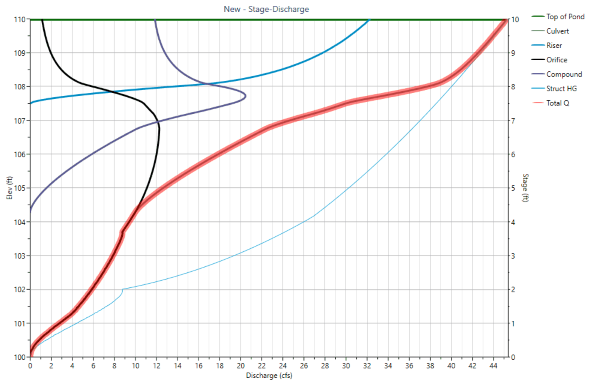This is a stage-discharge curve for a multi-stage structure. Notice how the flows from the orifice and weir tail back as the culvert begins to control the outflow. The riser is fully submerged at elevation 108.50.

## Culverts & Orifices

The standard orifice equation is used for culverts and orifices.### Inlet Control (culverts and orifices)

Q = discharge (cfs)
A = actual flow area (net area when using restrictor plate) (sqft)
h(i) = distance between the inlet water surface and the centroid of the culvert or orifice barrel (1/2 flow depth during partial flow) (ft)
Nb = number of barrels or orifices
Co = orifice coefficient
k = 1

### Outlet Control (culverts)

Q = discharge (cfs)
A = actual flow area (sqft)
h(o) = distance between the upstream
and downstream water surface
Nb = Number of barrels
Co = 1
k = 1.5 + [(29n2L)/R1.33]

Where:
n = Manning’s n-value
L = culvert length (ft)
R = area/wetted perimeter (ft)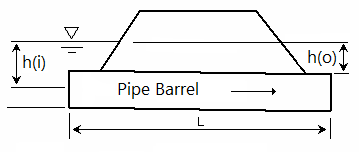During the calculation process, both inlet and outlet control are evaluated. Under inlet control, the discharge depends on the barrel shape, cross-sectional area of the pipe and inlet edge. Under outlet control, the discharge depends on the slope, length and roughness of the barrel. Under outlet control, flow can enter the structure at a faster rate than it can exit. Under Inlet control, it’s harder for water to enter the pipe than exit.

### Culvert Velocity

The culvert velocity is reported on the Pond Outlets Table next to the Culvert’s Q. This velocity is based on the associated Q and using Normal Depth as computed by Manning’s equation. Velocity = Q / A, where A is the cross-sectional area of Normal Depth. This velocity provides a more useful metric as it pertains to the Culvert’s normal flow at the downstream end or exit which can be used for scour considerations.

### Tailwater

When a tailwater (TW) elevation has been entered, it is compared the pond stage and computes a tailwater head, hTW. If this head is less than the head computed as above (h), then h = hTW.

### Perforated Riser

This is a special kind of orifice structure as shown below which contains a series of same-sized holes all within a vertical height. This outlet device can take the shape of a single multi-holed plate or an independent riser.

Orifice Plates for Water Quality Volume (WQv) Recommendations

A study performed by the Urban Drainage and Flood Control District, Denver, CO in 2016 resulted in the recommendation of limiting the number of water quality orifices to no more than three. When the number of orifices is limited to three (as opposed to the traditional three per foot of depth), the size of each orifice becomes larger and therefore less prone to clogging.

These orifices should spaced at stage zero, H/3, and 2H/3, where H is the maximum ponding depth of the water quality capture volume (WQv). For example, if the WQv volume produces a depth of 3 feet in your pond, you will want three holes. One at the invert (Stage 0.00); one at Stage 3 / 3 = 1.00; and the third at Stage 6 / 3 = 2.00. The Height value you will enter will be 2.00 plus the hole Rise in feet (meters).The following formula by McEnroe, 1988 is used to estimate the outflow.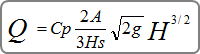Where:
Q = discharge (cfs)
Cp = 0.61
A = cross-sectional area of all the holes (sqft)
Hs = height (ft)
H = distance between water surface and the Invert Elevation (ft)

## WeirsHydrology Studio uses a standard weir equation for Rectangular, Compound, Cipoletti, Broad Crested & Circular or Box Riser structures.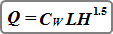Where:
Q = discharge over weir (cfs)
L = length of the weir crest (ft)
H = distance between water surface and the crest (ft)
Cw = weir coefficient, typically 3.33

### Broad Crested with Sloped Sides

Total flow over broad crested weirs with side slopes is computed using the standard weir equation as shown above, plus two times the flow given from the following equation.Where:
Q = discharge over side-sloped portion of weir (cfs)
Z = side slope (Z horizontal to 1 vertical) of the weir crest
H = distance between water surface and the crest (ft)
Cw = weir coefficient, typically 3.33

### V-notch

V-notch weirs are computed using this equation: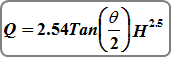Where: Q = discharge over weir (cfs)
Θ = angle of v-notch (degrees)
H = head on apex of v-notch (ft)

Rectangular, V-notch & Cipoletti weirs can be affected by submergence, i.e., when the tailwater rises above the weir’s crest.The equation for the reduction in flow is: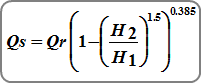Where:
Qs = submerged flow (cfs)
Qr = unsubmerged flow from standard weir equations
H1 = upstream head above crest (ft)
H2 = downstream head above crest (ft)

An “s” is added as a suffix to numbers displayed in the Stage-Discharge table to indicate when flows have been adjusted for submergence.

Not applicable to Riser weir flow.